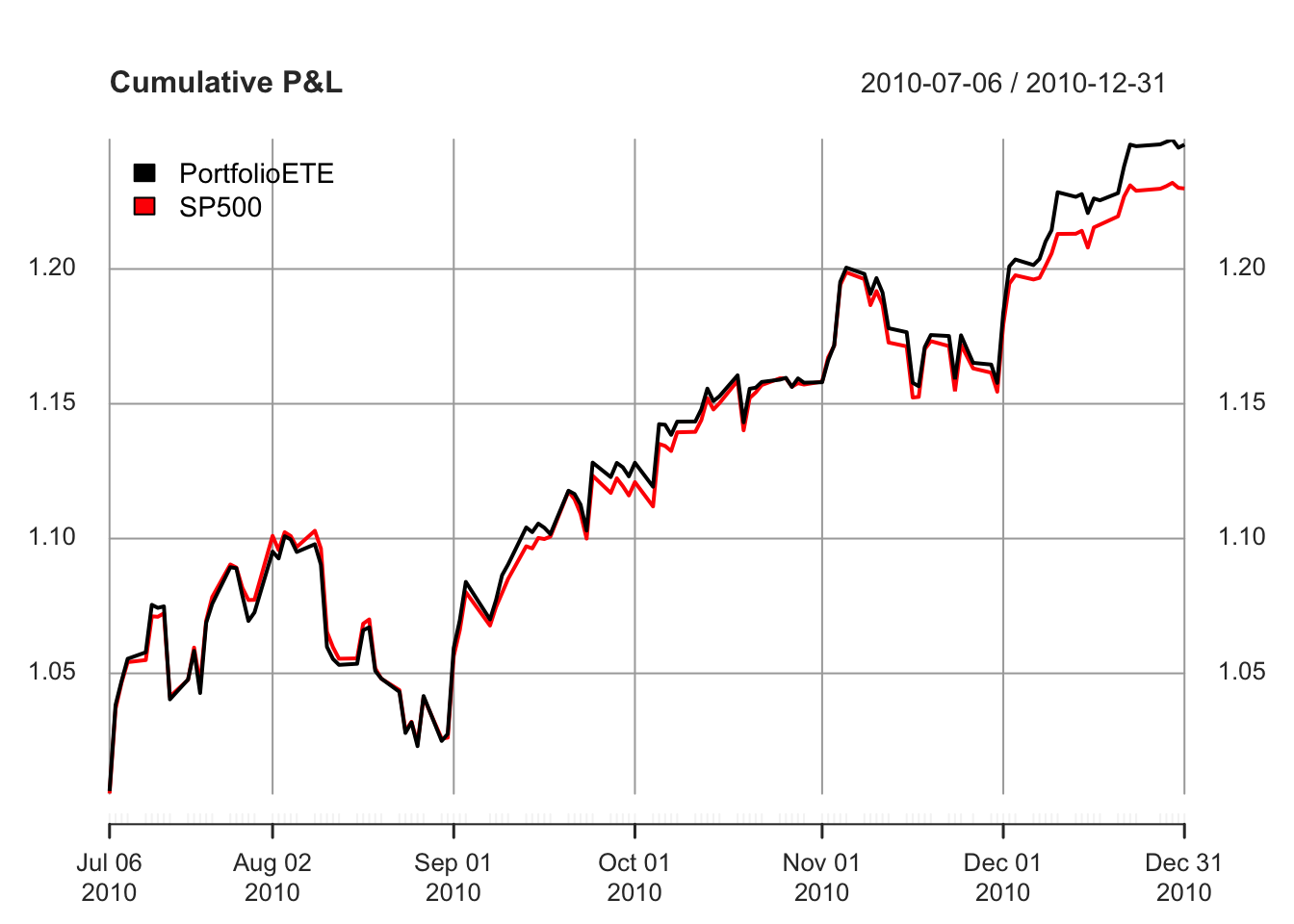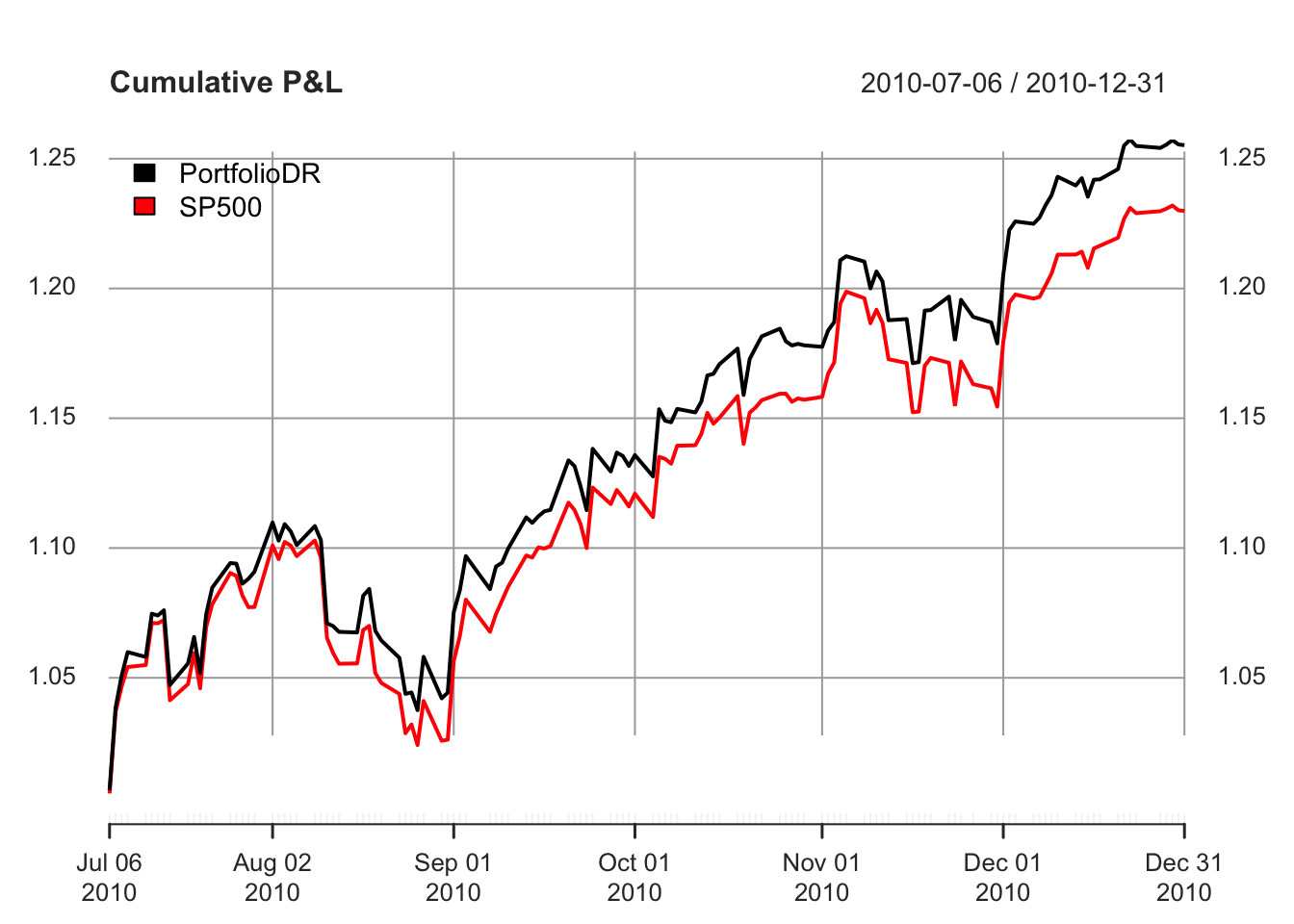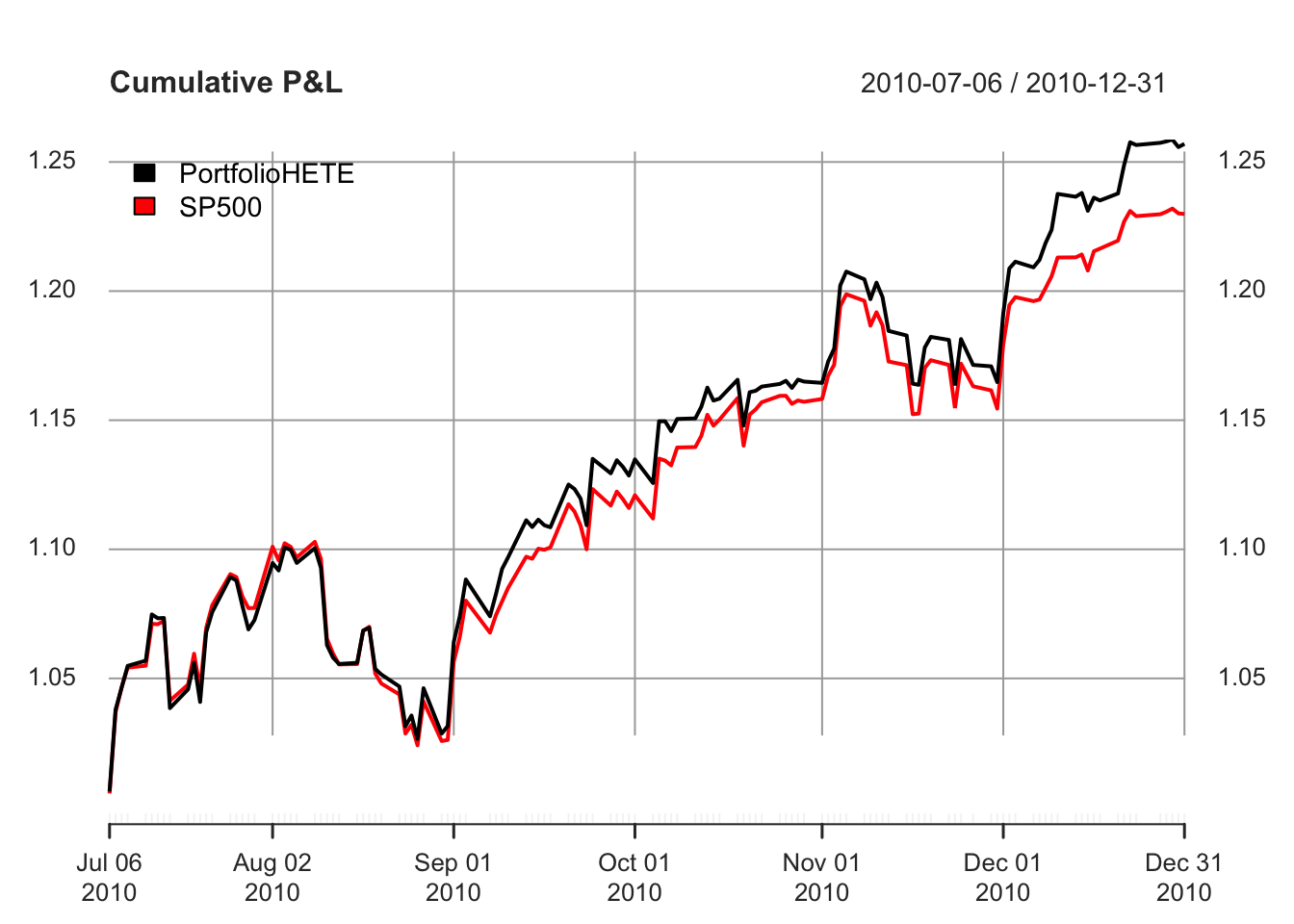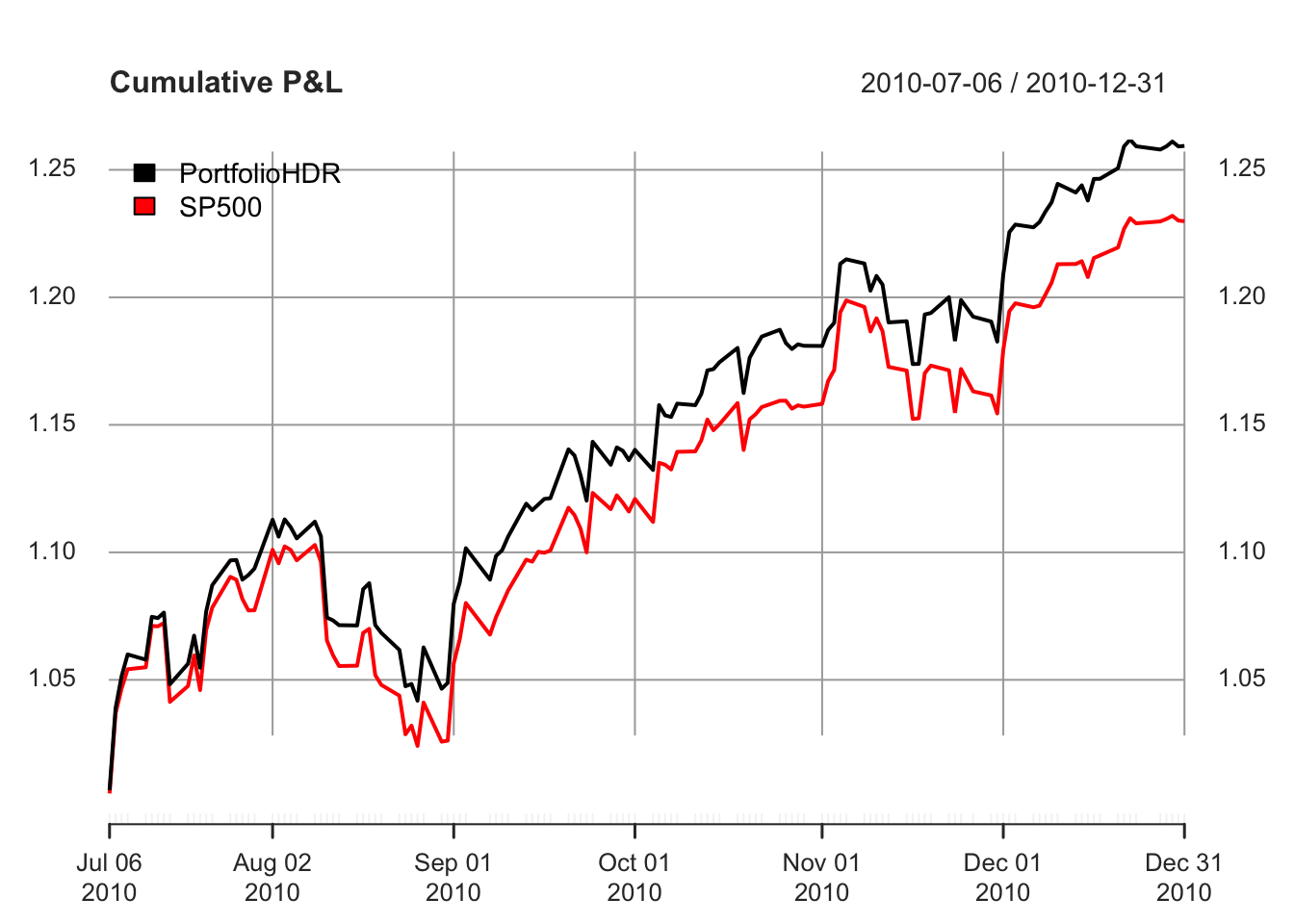# Design of Portfolio of Stocks to Track an Index

### 2018-09-01

This vignette illustrates the design of sparse portfolios that aim to track a financial index with the package sparseIndexTracking (with a comparison with other packages) and gives a description of the algorithms used.

# Comparison with other packages

There are currently no other R packages for index tracking. In paper  and monograph , a detailed comparison in terms of execution speed and performance is made with the mixed-integer quadratic programming (MIQP) solver Gurobi (for which R has an interface via package ROI and plugin ROI.plugin.gurobi).

# Usage of the package

We start by loading the package and real data of the index S&P 500 and its underlying assets:

library(sparseIndexTracking)
library(xts)
data(INDEX_2010)

The data INDEX_2010 contains a list with two xts objects:

1. X: A $$T\times N$$ xts with the daily linear returns of the $$N$$ assets that were in the index during the year 2010 (total $$T$$ trading days)
2. SP500: A $$T\times 1$$ xts with the daily linear returns of the index S&P 500 during the same period.

Note that we use xts objects just for illustration purposes. The function spIndexTrack() can also be invoked passing simple data arrays or dataframes.

Based on the above quantities we create a training window, which we will use to create our portfolios, and a testing window, which will be used to assess the performance of the designed portfolios. For simplicity, here we consider the first six (trading) months of the dataset (~126 days) as the training window, and the subsequent six months as the testing window:

X_train <- INDEX_2010$X[1:126] X_test <- INDEX_2010$X[127:252]
r_train <- INDEX_2010$SP500[1:126] r_test <- INDEX_2010$SP500[127:252]

Now, we use the four modes (four available tracking errors) of the spIndexTrack() algorithm to design our portfolios:

# ETE
w_ete <- spIndexTrack(X_train, r_train, lambda = 1e-7, u = 0.5, measure = 'ete')
cat('Number of assets used:', sum(w_ete > 1e-6))
#> Number of assets used: 45

# DR
w_dr <- spIndexTrack(X_train, r_train, lambda = 2e-8, u = 0.5, measure = 'dr')
cat('Number of assets used:', sum(w_dr > 1e-6))
#> Number of assets used: 42

# HETE
w_hete <- spIndexTrack(X_train, r_train, lambda = 8e-8, u = 0.5, measure = 'hete', hub = 0.05)
cat('Number of assets used:', sum(w_hete > 1e-6))
#> Number of assets used: 44

# HDR
w_hdr <- spIndexTrack(X_train, r_train, lambda = 2e-8, u = 0.5, measure = 'hdr', hub = 0.05)
cat('Number of assets used:', sum(w_hdr > 1e-6))
#> Number of assets used: 43

Finally, we plot the actual value of the index in the testing window in comparison with the values of the designed portfolios:

plot(cbind("PortfolioETE" = cumprod(1 + X_test %*% w_ete), cumprod(1 + r_test)),
legend.loc = "topleft", main = "Cumulative P&L")plot(cbind("PortfolioDR" = cumprod(1 + X_test %*% w_dr), cumprod(1 + r_test)),
legend.loc = "topleft", main = "Cumulative P&L")plot(cbind("PortfolioHETE" = cumprod(1 + X_test %*% w_hete), cumprod(1 + r_test)),
legend.loc = "topleft", main = "Cumulative P&L")plot(cbind("PortfolioHDR" = cumprod(1 + X_test %*% w_hdr), cumprod(1 + r_test)),
legend.loc = "topleft", main = "Cumulative P&L")In the above examples we used a single training and testing window. In practice, we need to perform this task sequentially for many windows in order to assess an algorithm or to distinguish the differences between the various tracking errors.

Ideally, the ETE and HETE portfolios should have Excess P&L close to zero since their purpose is to track as closely as possible the index, whereas the DR and HDR portfolios should have a positive Excess P&L since their purpose is to beat the index. Finally, Huber should shine in periods of high volatility where many extreme returns are observed (like the great recession).

All of the above can be observed in Figures 1 - 3 where we applied the four modes of the algorithm in the index S&P 500 considering three different periods. All the constructed portfolios consist of 40 assets, the training and testing windows were set to 6 months and 1 month, respectively, while monthly returns were used. The upper plot of each period (Normalized P&L) shows the wealth of the index and the four portfolios, which are normalized to the index value each time they are rebalanced. The lower plot of each period (Excess P&L) shows the cumulative difference of the portfolios and the index due to normalization, i.e., it is equivalent to a second account that keeps track of our excess profits or losses.